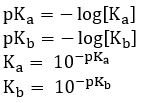# 18.2 Calculations involving acids and bases

### The strengths of acids and the acid dissociation constant

• Strong acids and bases:
• Since strong acids are assumed to completely dissociate in water, the concentration of each of the two ions produced is the same as the initial concentration of a strong monoprotic acid (one hydrogen)• Weak acids and bases:
• Since these do not complete dissociate, the concentration of products is not the same
• The way to solve for concentrations is using the equilibrium expression
• Acid dissociation constant:
• Base dissociation constant:

### Calculating Ka and Kb

1. Write out the complete reaction
2. Complete an ICE table
3. Determine Ka or Kb

### The temperature dependence of Kw

• Kw is defined only at 25°C
• The ionization of water is endothermic, so as temperature increases, equilibrium shifts right
• Increase in concentration of H+ results in decrease in pH

### pKa and pKb

• The pH scale can be applied to Ka and Kb to get pKa and pKb